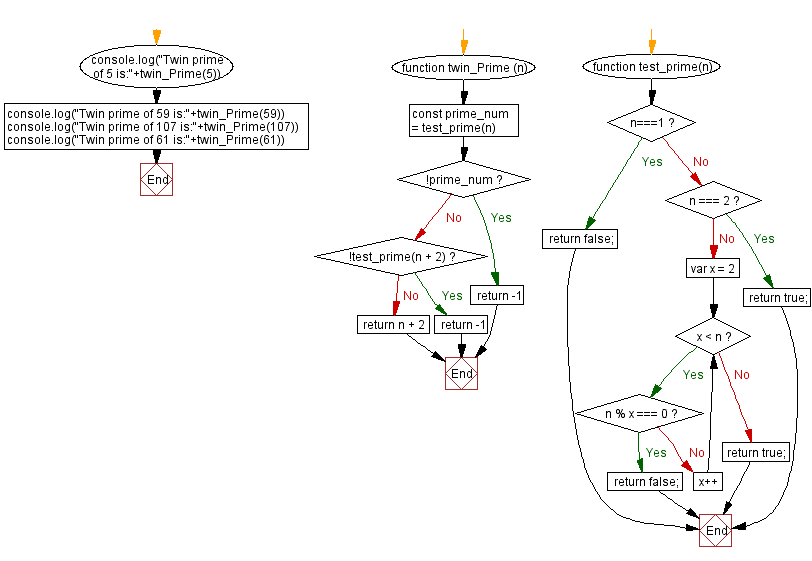# JavaScript: Twin prime of a prime number

## JavaScript Math: Exercise-66 with Solution

Write a JavaScript program to find the 'twin prime' of a given prime number.

From Wikipedia,
A twin prime is a prime number that is either 2 less or 2 more than another prime number-for example, either member of the twin prime pair (41, 43). In other words, a twin prime is a prime that has a prime gap of two. Sometimes the term twin prime is used for a pair of twin primes; an alternative name for this is prime twin or prime pair.
Usually the pair (2, 3) is not considered to be a pair of twin primes. Since 2 is the only even prime, this pair is the only pair of prime numbers that differ by one; thus twin primes are as closely spaced as possible for any other two primes.
The first few twin prime pairs are:
(3, 5), (5, 7), (11, 13), (17, 19), (29, 31), (41, 43), (59, 61), (71, 73), (101, 103), (107, 109), (137, 139), ....

Return value: Either the twin, or -1 if n or n + 2 is not prime number.

Sample Data:
Twin prime of 5 is:7
Twin prime of 59 is:61
Twin prime of 107 is:109
Twin prime of 61 is:-1

Sample Solution:

HTML Code:

``````<!DOCTYPE html>
<html>
<meta charset="utf-8">
<title>JavaScript function to find the twin prime number</title>
<body>

</body>
</html>
```
```

JavaScript Code:

``````function twin_Prime (n) {
const prime_num = test_prime(n)

if (!prime_num) {
return -1
}
if (!test_prime(n + 2)) {
return -1
}

return n + 2
}
function test_prime(n)
{
if (n===1)
{
return false;
}
else if(n === 2)
{
return true;
}
else
{
for(var x = 2; x < n; x++)
{
if(n % x === 0)
{
return false;
}
}
return true;
}
}
console.log("Twin prime of 5 is:"+twin_Prime(5))
console.log("Twin prime of 59 is:"+twin_Prime(59))
console.log("Twin prime of 107 is:"+twin_Prime(107))
console.log("Twin prime of 61 is:"+twin_Prime(61))
```
```

Sample Output:

```"Twin prime of 5 is:7"
"Twin prime of 59 is:61"
"Twin prime of 107 is:109"
"Twin prime of 61 is:-1"
```

Flowchart:Live Demo:

See the Pen javascript-math-exercise-66 by w3resource (@w3resource) on CodePen.

Improve this sample solution and post your code through Disqus

What is the difficulty level of this exercise?

Test your Programming skills with w3resource's quiz.

﻿